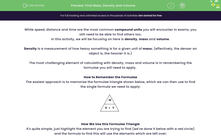# Find Mass, Density and Volume

In this worksheet, students will practise using the Mass, Density, Volume formulae pyramid to identify the correct single formula to apply to find a missing metric.Key stage:  KS 4

Year:  GCSE

GCSE Subjects:   Maths

GCSE Boards:   AQA, Eduqas, Pearson Edexcel, OCR,

Popular topics:   Geometry worksheets

Difficulty level:#### Worksheet Overview

While speed, distance and time are the most common compound units you will encounter in exams, you still need to be able to find others too.

In this activity, we will be focusing on here is density, mass and volume.

Density is a measurement of how heavy something is for a given unit of mass; (effectively, the denser an object is, the heavier it is.)

The most challenging element of calculating with density, mass and volume is in remembering the formulae you will need to apply.

How to Remember the Formulae

The easiest approach is to memorise the formulae triangle shown below, which we can then use to find the single formula we need to apply:How We Use this Formulae Triangle

It's quite simple, just highlight the element you are trying to find (we've done it below with a red circle) and the formula to find this will use the elements which are left over:Let's look at these formulae in action now in some examples.

e.g. Find the volume of an object that has a density of 20 g/cm3 and a mass of 500 g.

The first step in these calculations is always to decide which element we are trying to find.

For this question, we are directly asked for the volume, so we need to use:

V = M ÷ D

Now we can simply plug our numbers into this formula:

V = 500 ÷ 20

The last question that we need to ask ourselves is what units of measurement we need to use.

The trick here is that they are always the same as one of the units provided in the question.

The density here is given in g/cm3, so our volume must be cm3.

So Distance = 25 cm3

e.g. An object has a volume of 75 cm3 and a mass of 15 g. Find the average density of the object.

Once again, we need to decide which formula.

Don't worry that it asks for average density here, we can still use the same formula:

D = M ÷ V

Plugging in our numbers gives us:

D = 15 ÷ 75 = 0.2

Our units are grams per cm3 (remember, the units always are in the question).

So Density = 0.2 g/cm3

In this activity, we will use the Mass, Density, Volume formulae pyramid to identify the correct single formula to apply to find a missing metric using the method demonstrated above.

### What is EdPlace?

We're your National Curriculum aligned online education content provider helping each child succeed in English, maths and science from year 1 to GCSE. With an EdPlace account you’ll be able to track and measure progress, helping each child achieve their best. We build confidence and attainment by personalising each child’s learning at a level that suits them.

Get started